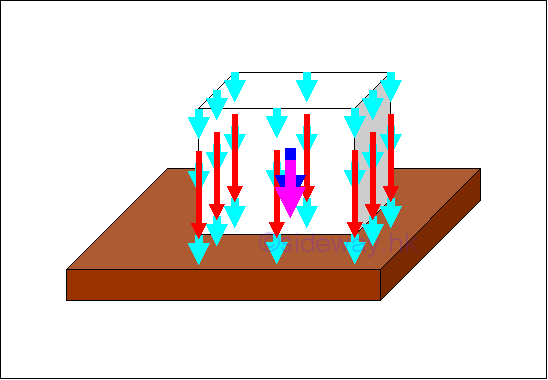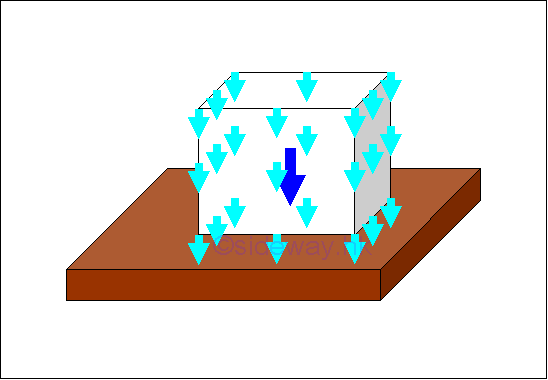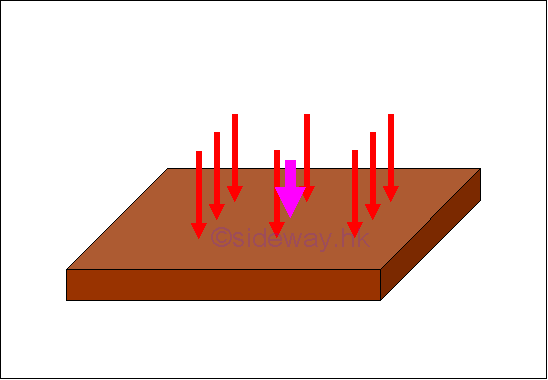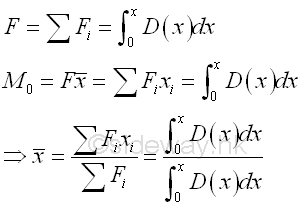output.to from Sideway
Mechanics: StaticsMechanics Dynamics

Draft for Information Only

# Content

``` Distributed Force   Body Force:  Distributed Load:```

# Distributed ForceA force acting on a point along a single line in space is a concentrated force. A concentrated force is the mathematical idealization of forces in practical problems, in which all forces are either body forces acting over a volume or surface forces acting over an area. These types of forces are called distributed forces which are either distributed over a specific volume or spread out over a specific area. Through system of forces transformation, the distributed forces can be represented by a concentrated force.

## Body Force:In static mechanic, a body force is the attraction force exerted by the earth on a rigid body. The attraction force is called the force of gravity or in general, the weight of the rigid body. The force of gravity acts on each of the particles of the entire body. Therefore the gravitational forces of a body can be represented by numbers of small gravitational forces distributed over the body volume. In mathematical idealization, the gravitational force of the body is represented by a resultant force acting on the center of gravity of the body as the weight of the body.

The center of gravity of a body is related to the gravitation attraction, density and shape of the body. For an uniform gravitational force field, the center of mass of a body is equal to the center of gravity of a body. And in practical problems, the center of gravity is sometimes also refered to the center of mass. For a homogeneous body with constant mass density, the centroid of the shape of a body is equal to the center of gravity. ImplySimilarly, many mechanical structures are subjected to distributed loads spread out over a specific area rather than concentrated loads in static mechanic. However, an equivalent normal concentrated force is often used to represent the applied distributed loads spread out over a specific area when solving practical problems.

For an uniform distributed load, a load of constant magnitude is applied over a specific area. The resultant of these distributed loads can be represented by a normal concentrated load with magnitude equals to the sum of the distributed loads or the product of uniform distributed load per unit area and the applied area, acting on the center of gravity or center of mass, or center of centroid of the applied area of applied load.

In general, the magnitude of the equivalent normal concentrated load for the resultant force of a distributed load is equal to the summation of the all distributed forces or the area under the function of the distributed load. And the applying position of the equivalent normal concentrated load can also determined by taking moment about a pont or an axis as in body force. For example, a distribution load of load distribution function, D(x) spread on a beam along x axis, implyID: 120300021 Last Updated: 3/28/2012 Revision: 0 Ref:References

1. I.C. Jong; B.G. rogers, 1991, Engineering Mechanics: Statics and Dynamics
2. F.P. Beer; E.R. Johnston,Jr.; E.R. Eisenberg, 2004, Vector Mechanics for Engineers: StaticsHome 5

Management

HBR 3

Information

Recreation

Culture

Chinese 1097

English 339

Computer

Hardware 249

Software

Application 213

Latex 52

Manim 205

KB 1

Numeric 19

Programming

Web 289

Unicode 504

HTML 66

CSS 65

SVG 46

ASP.NET 270

OS 429

Python 72

Knowledge

Mathematics

Algebra 84

Geometry 34

Calculus 67

Engineering

Mechanical

Rigid Bodies

Statics 92

Dynamics 37

Control

Natural Sciences

Electric 27